# Help with 747 aircraft.cfgSmailey112 Guest

Hey, I tried to add some now repaints for my 747, and when I was on the b747_400 I clicked on the aircraft.cfg.

What happend next, was that some of the things on the aircraft cfg dissapered, and now my 747 on FSX is bugged. It falls trough the ground, and I can't use it.

So if someone would help me, by looking at there b747_400/aircraft.cfg for me, and copy it on this forum, so I could get the right one back.

This would fix all my problems.

Thank you for all help/tips,
Smailey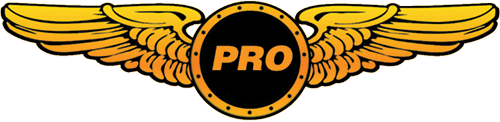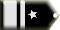Tailhook Chief Captain

Here you are:

[fltsim.0]
title=Boeing 747-400 Paint1
sim=Boeing747-400
model=
panel=
sound=
texture=1
kb_checklists=Boeing747-400_check
kb_reference=Boeing747-400_ref
atc_id=N747K
atc_airline=Boeing
atc_flight_number=1123
atc_heavy=1
ui_manufacturer="Boeing"
ui_type="747-400"
ui_variation="Boeing livery"
ui_typerole="Commercial Airliner"
ui_createdby="Microsoft Corporation"
description="More than 30 years ago, the 747 made its first trip from New York to London. Since then, it's become the standard by which other large passenger jets are judged. Its size, range, speed and capacity were then, and are now, the best in its class."

[fltsim.1]
title=Boeing 747-400 Paint2
sim=Boeing747-400
model=
panel=
sound=
texture=2
kb_checklists=Boeing747-400_check
kb_reference=Boeing747-400_ref
atc_id=N747I
atc_airline=World Travel
atc_flight_number=1123
atc_heavy=1
ui_manufacturer="Boeing"
ui_type="747-400"
ui_variation="World Travel Airlines"
ui_typerole="Commercial Airliner"
ui_createdby="Microsoft Corporation"
description="More than 30 years ago, the 747 made its first trip from New York to London. Since then, it's become the standard by which other large passenger jets are judged. Its size, range, speed and capacity were then, and are now, the best in its class."

[fltsim.2]
title=Boeing 747-400 Paint4
sim=Boeing747-400
model=
panel=
sound=
texture=4
kb_checklists=Boeing747-400_check
kb_reference=Boeing747-400_ref
atc_id=N747P
atc_airline=Orbit
atc_flight_number=1123
atc_heavy=1
ui_manufacturer="Boeing"
ui_type="747-400"
ui_variation="Orbit Airlines"
ui_typerole="Commercial Airliner"
ui_createdby="Microsoft Corporation"
description="More than 30 years ago, the 747 made its first trip from New York to London. Since then, it's become the standard by which other large passenger jets are judged. Its size, range, speed and capacity were then, and are now, the best in its class."

[fltsim.3]
title=Boeing 747-400 Paint5
sim=Boeing747-400
model=
panel=
sound=
texture=5
kb_checklists=Boeing747-400_check
kb_reference=Boeing747-400_ref
atc_id=N747I
atc_airline=Pacifica
atc_flight_number=1123
atc_heavy=1
ui_manufacturer="Boeing"
ui_type="747-400"
ui_variation="Pacifica Airlines"
ui_typerole="Commercial Airliner"
ui_createdby="Microsoft Corporation"
description="More than 30 years ago, the 747 made its first trip from New York to London. Since then, it's become the standard by which other large passenger jets are judged. Its size, range, speed and capacity were then, and are now, the best in its class."

[fltsim.4]
title=Boeing 747-400 Paint8
sim=Boeing747-400
model=
panel=
sound=
texture=8
kb_checklists=Boeing747-400_check
kb_reference=Boeing747-400_ref
atc_id=N747I
atc_airline=Global Freight
atc_flight_number=1123
atc_heavy=1
atc_parking_types=CARGO
ui_manufacturer="Boeing"
ui_type="747-400"
ui_variation="Global Freightways"
ui_typerole="Commercial Airliner"
ui_createdby="Microsoft Corporation"
description="More than 30 years ago, the 747 made its first trip from New York to London. Since then, it's become the standard by which other large passenger jets are judged. Its size, range, speed and capacity were then, and are now, the best in its class."

[fltsim.5]
title=Boeing 747-400 Paint9
sim=Boeing747-400
model=
panel=
sound=
texture=9
kb_checklists=Boeing747-400_check
kb_reference=Boeing747-400_ref
atc_id=N747I
atc_airline=Mothball
atc_flight_number=1123
atc_heavy=1
ui_manufacturer="Boeing"
ui_type="747-400"
ui_variation="Decommissioned"
ui_typerole="Commercial Airliner"
ui_createdby="Microsoft Corporation"
description="More than 30 years ago, the 747 made its first trip from New York to London. Since then, it's become the standard by which other large passenger jets are judged. Its size, range, speed and capacity were then, and are now, the best in its class."

[fltsim.6]
title=Boeing 747-400
sim=Boeing747-400
model=
panel=
sound=
texture=
kb_checklists=Boeing747-400_check
kb_reference=Boeing747-400_ref
atc_id=N747
atc_airline=
atc_flight_number=1123
atc_heavy=1
atc_parking_types=CARGO
ui_manufacturer="Boeing"
ui_type="747-400"
ui_variation="White"
ui_typerole="Commercial Airliner"
ui_createdby="Microsoft Corporation"
description="More than 30 years ago, the 747 made its first trip from New York to London. Since then, it's become the standard by which other large passenger jets are judged. Its size, range, speed and capacity were then, and are now, the best in its class."

[General]
atc_type=BOEING
atc_model=B744
editable=0
performance="Cruise Speed\n0.85 Mach 565 mph 910 km\/h\n\nEngine options\nPratt & Whitney PW4062\nRolls-Royce RB211-524H\nGeneral Electric CF6-80C2B5F\n\nMaximum Range\n7,325 nm 13,570 km\n\nMaximum Certified Operating Altitude 45,100 ft 13,747 m\n\nFuel Capacity\n57,285 gal 216,840 L\n\nBasic Empty Weight\n394,088 lb 178,755 kg\n\nMax Gross Weight 875,000 lb 396,893 kg\n\nLength\n231 ft, 10 in 70.6 m\n\nWingspan\n211 ft, 5 in 64.4 m\n\nHeight\n63 ft, 8 in 19.4 m\n\nSeating Typical 3-class configuration - 416\nTypical 2-class configuration - 524"
Category = airplane

Title = "Right Side Window"
Guid = {54F54B8A-3EC2-2D4E-8D10-B8F9D0F16ACC}
Description = View of the right wing from the passenger cabin
Origin = Center
SnapPbhReturn = FALSE
PanPbhReturn = FALSE
Track = None
ShowAxis = FALSE
AllowZoom = TRUE
InitialZoom = 1.0
ShowWeather = Yes
InitialXyz = 5.5, 0.75, -13
InitialPbh = 0, 0, 95
Category=Aircraft
MomentumEffect=TRUE
ClipMode=Minimum

Title = "Left Side Window"
Guid = {0ae3f864-da10-4e5a-977c-b9bba47d6f7a}
Description = View of the left wing from the passenger cabin
Origin = Center
SnapPbhReturn = FALSE
PanPbhReturn = FALSE
Track = None
ShowAxis = FALSE
AllowZoom = TRUE
InitialZoom = 1.0
ShowWeather = Yes
InitialXyz = -5.5, 0.75, -13
InitialPbh = 0, 0, 265
Category=Aircraft
MomentumEffect=TRUE
ClipMode=Minimum

Title = "Landing Gear"
Description = View of the landing gear and left wing inboard flaps
Origin = Center
SnapPbhReturn = FALSE
PanPbhReturn = FALSE
Track = None
ShowAxis = FALSE
AllowZoom = TRUE
InitialZoom = .58
ShowWeather = Yes
InitialXyz = -11, -4.1, -16
InitialPbh = 0, 0, 44
Category=Aircraft
MomentumEffect=TRUE
ClipMode=Minimum

Title = "Tail"
Guid = {5c1df273-034b-4e7f-953a-9d5e26f1646c}
Description = Looking forward from behind the aircraft
Origin = Center
SnapPbhReturn = FALSE
PanPbhReturn = FALSE
Track = None
ShowAxis = FALSE
AllowZoom = TRUE
InitialZoom = .75
ShowWeather = Yes
InitialXyz = 0, 9.0, -80.0
InitialPbh = 5, 0, 0
Category=Aircraft
MomentumEffect=TRUE
ClipMode=Minimum

Title = "Right Wing"
Description = View from the right wing tip looking at the cockpit
Origin = Center
SnapPbhReturn = FALSE
PanPbhReturn = FALSE
Track = None
ShowAxis = FALSE
AllowZoom = TRUE
InitialZoom = 0.5
ShowWeather = Yes
InitialXyz = 40.8, 1.4, 4.0
InitialPbh = 6, 0, 270
Category=Aircraft
MomentumEffect = FALSE
ClipMode=Minimum

Title = "Left Wing"
Guid = {f25dfa2e-fd2e-4b89-81e2-0575cbcb60de}
Description = View from the left wing tip looking at the cockpit
Origin = Center
SnapPbhReturn = FALSE
PanPbhReturn = FALSE
Track = None
ShowAxis = FALSE
AllowZoom = TRUE
InitialZoom = 0.5
ShowWeather = Yes
InitialXyz = -40.8, 1.4, 4.0
InitialPbh = 6, 0, 90
Category=Aircraft
MomentumEffect = No
ClipMode=Minimum

Title = "Right Seat"
Guid = {8ff6c134-098d-409f-baec-caba3f683f98}
Origin = Virtual Cockpit
MomentumEffect = YES
SnapPbhReturn = False
PanPbhReturn = False
Track = None
ShowAxis = YES
AllowZoom = TRUE
InitialZoom = .75
SmoothZoomTime = 2.0
ZoomPanScalar = 1.0
ShowWeather = Yes
ShowLensFlare=FALSE
Category = Cockpit
PitchPanRate=20
InitialXyz=1.25, 0.0, 0.0
InitialPbh=0, 0, 0

Guid = {195EAB58-9E4A-2E2A-A34C-A8D9D948F078}
Origin = Virtual Cockpit
MomentumEffect = No
SnapPbhReturn = False
PanPbhReturn = False
Track = None
ShowAxis = FALSE
AllowZoom = TRUE
InitialZoom = .75
SmoothZoomTime = 2.0
ZoomPanScalar = 1.0
ShowWeather = Yes
ShowLensFlare=FALSE
Category = Cockpit
PitchPanRate=20
InitialXyz=0.6, 0.30, -0.05
InitialPbh=85, 0, 0

Guid = {4ca337db-ae88-4c5f-96ea-ddebbd2a86fe}
Origin = Virtual Cockpit
MomentumEffect = No
SnapPbhReturn = False
PanPbhReturn = False
Track = None
ShowAxis = FALSE
AllowZoom = TRUE
InitialZoom = 0.8
SmoothZoomTime = 2.0
ZoomPanScalar = 1.0
ShowWeather = Yes
ShowLensFlare=FALSE
Category = Cockpit
PitchPanRate=20
InitialXyz=0.635, -0.58, -0.2
InitialPbh=-55.0, 0, 0

Title = "Engine Controls"
Guid = {195EAB58-9E4A-3E2A-A34C-A8D9D948F078}
Origin = Virtual Cockpit
MomentumEffect = No
SnapPbhReturn = False
PanPbhReturn = False
Track = None
ShowAxis = FALSE
AllowZoom = TRUE
InitialZoom = 1.0
SmoothZoomTime = 2.0
ZoomPanScalar = 1.0
ShowWeather = Yes
ShowLensFlare=FALSE
Category = Cockpit
PitchPanRate=20
InitialXyz=0.6, 0.3, 0
InitialPbh=59.5, 0, 0

[pitot_static]
vertical_speed_time_constant = 1 //Increasing this value will cause a more instantaneous reaction in the VSI
pitot_heat = 1.0 //Scalar on heat effectiveness, 0 = not available

[WEIGHT_AND_BALANCE]
max_gross_weight = 875000 // (pounds)
empty_weight = 394088 // (pounds)

reference_datum_position = 83.5, 0, 0 // (feet) distance from FlightSim Reference position: (1/4 chord, centerline, waterline)
empty_weight_CG_position = -90.5, 0, 0 // (feet) longitudinal, lateral, vertical distance from specified datum

max_number_of_stations = 50

station_load.0 = 170.0, -19.0, -2.0, 8.0 //Weight (lbs), longitudinal, lateral, vertical positions from datum (feet)
station_load.1 = 170.0, -19.0, 2.0, 8.0 //Weight (lbs), longitudinal, lateral, vertical positions from datum (feet)
station_load.2 = 1700.0, -83.5, 0.0, 0.0 //Weight (lbs), longitudinal, lateral, vertical positions from datum (feet)
station_load.3 = 5440.0, -27.0, 0.0, 0.0 //Weight (lbs), longitudinal, lateral, vertical positions from datum (feet)
station_load.4 = 5780.0, -65.0, 0.0, 0.0 //Weight (lbs), longitudinal, lateral, vertical positions from datum (feet)
station_load.5 = 7140.0, -50.0, 0.0, 8.0 //Weight (lbs), longitudinal, lateral, vertical positions from datum (feet)
station_load.6 = 10710.0, -91.0, 0.0, 0.0 //Weight (lbs), longitudinal, lateral, vertical positions from datum (feet)
station_load.7 = 18020.0, -119.0, 0.0, 0.0 //Weight (lbs), longitudinal, lateral, vertical positions from datum (feet)
station_load.8 = 23800.0, -160.0, 0.0, 0.0 //Weight (lbs), longitudinal, lateral, vertical positions from datum (feet)
station_load.9 = 18000.0, -52.0, 0.0, -6.0 //Weight (lbs), longitudinal, lateral, vertical positions from datum (feet)
station_load.10 = 6000.0, -123.0, 0.0, -6.0 //Weight (lbs), longitudinal, lateral, vertical positions from datum (feet)

station_name.0 = "Pilot"
station_name.1 = "Co-Pilot"
station_name.2 = "Crew"
station_name.3 = "First Class"
station_name.5 = "Upper Deck"
station_name.6 = "Coach 1-7"
station_name.7 = "Coach 8-18"
station_name.8 = "Coach 19-34"
station_name.9 = "Forward Baggage"
station_name.10 = "Aft Baggage"

;Moments of Inertia
empty_weight_roll_MOI = 13352310
empty_weight_pitch_MOI = 24223159
empty_weight_yaw_MOI = 39531785
empty_weight_coupled_MOI = 0

[fuel]
LeftMain = -96.0, -28.0, -4.0, 12546.0, 0.0 //Longitudinal (feet), Lateral (feet), Vertical (feet), Usable(gallons), Unusable (gallons)
RightMain = -96.0, 28.0, -4.0, 12546.0, 0.0 //Longitudinal (feet), Lateral (feet), Vertical (feet), Usable(gallons), Unusable (gallons)
LeftAux = -112.0, -36.5, -3.0, 4482.0, 0.0 //Longitudinal (feet), Lateral (feet), Vertical (feet), Usable(gallons), Unusable (gallons)
RightAux = -112.0, 36.5, -3.0, 4482.0, 0.0 //Longitudinal (feet), Lateral (feet), Vertical (feet), Usable(gallons), Unusable (gallons)
LeftTip = -130.0, -73.0, 0.0, 1322.0, 0.0 //Longitudinal (feet), Lateral (feet), Vertical (feet), Usable(gallons), Unusable (gallons)
RightTip = -130.0, 73.0, 0.0, 1322.0, 0.0 //Longitudinal (feet), Lateral (feet), Vertical (feet), Usable(gallons), Unusable (gallons)
Center1 = -83.5, 0.0, -7.0, 17164.0, 0.0 //Longitudinal (feet), Lateral (feet), Vertical (feet), Usable(gallons), Unusable (gallons)
Center2 = -193.5, 0.0, 6.0, 3300.0, 0.0 //Longitudinal (feet), Lateral (feet), Vertical (feet), Usable(gallons), Unusable (gallons)

fuel_type = 2 //Fuel type: 1 = Avgas, 2 = JetA
number_of_tank_selectors = 1
electric_pump=1
fuel_dump_rate = 0.0167 //Percent of max quantity per second, i.e. about 1 minute for full fuel

[airplane_geometry]
wing_area = 5825.0 //Square feet
wing_span = 211.4 //Feet
wing_root_chord = 48.8 //Feet
wing_dihedral = 7.0 //Degrees
wing_incidence = 2.0 //Degrees
wing_twist = -1.0 //Degrees
oswald_efficiency_factor= 0.68 //Measure of lift effeciency of wing
wing_winglets_flag = 1 //Are winglets available?
wing_sweep = 37.5 //Degrees, wing leading edge
wing_pos_apex_lon = -58.2 //Feet, longitudinal distance from reference point, negative going aft
wing_pos_apex_vert = -5 //Feet, vertical distance from reference point, positive going up
htail_area = 1470 //Square feet
htail_span = 72.8 //Feet
htail_pos_lon = -210.0 //Feet, longitudinal distance from reference point, negative going aft
htail_pos_vert = 0.0 //Feet, vertical distance from reference point, positive going up
htail_incidence = 0.0 //Degrees
htail_sweep = 37.5 //Degrees, horizontal tail leading edge
vtail_area = 1060 //Square feet
vtail_span = 37.1 //Feet, tip to body
vtail_sweep = 45.0 //Degrees, vertical tail leading edge
vtail_pos_lon = -198.5 //Feet, longitudinal distance from reference point, negative going aft
vtail_pos_vert = 26.1 //Feet, vertical distance from reference point, positive going up
elevator_area = 327 //Square feet
aileron_area = 225 //Square feet
rudder_area = 230 //Square feet
elevator_up_limit = 25 //Degrees
elevator_down_limit = 15 //Degrees
aileron_up_limit = 25 //Degrees
aileron_down_limit = 15 //Degrees
rudder_limit = 31.5 //Degrees
elevator_trim_limit = 20 //Degrees
spoiler_limit = 45 //Degrees
spoiler_extension_time = 2.0 //Seconds
spoilerons_available = 1 //Spoilerons Available?
aileron_to_spoileron_gain = 3 //Aileron to spoileron gain
min_ailerons_for_spoilerons = 10 //Degrees
min_flaps_for_spoilerons = 0 //Minimum flap handle position when spoilerons activate
auto_spoiler_available = 1
positive_g_limit_flaps_up = 4.0 //Design G load tolerance (positive, flaps up)
positive_g_limit_flaps_down= 3.0 //Design G load tolerance (positive, flaps down)
negative_g_limit_flaps_up = -3.0 //Design G load tolerance (negative, flaps up)
negative_g_limit_flaps_down= -2.0 //Design G load tolerance (negative, flaps down)

[Reference Speeds]
flaps_up_stall_speed = 140.0 //Knots True (KTAS)
full_flaps_stall_speed = 112.0 //Knots True (KTAS)
cruise_speed = 505.0 //Knots True (KTAS)
max_mach = 0.92
max_indicated_speed = 365.0 //Red line (KIAS)

[flaps.0]
type = 1 // 1 - tail, 2 - lead
span-outboard = 0.8 // 0.0 .. 1.0
extending-time = 25 // seconds
flaps-position.0 = 0 // degrees
flaps-position.1 = 1 // degrees
flaps-position.2 = 5 // degrees
flaps-position.3 = 10 // degrees
flaps-position.4 = 20 // degrees
flaps-position.5 = 25 // degrees
flaps-position.6 = 30 // degrees
damaging-speed = 200 // KIAS
blowout-speed = 250 // KIAS
lift_scalar = 0.7
drag_scalar = 0.9
pitch_scalar= 0.9
system_type = 1 //Hydraulic

[flaps.1]
type = 2 // 1 - tail, 2 - lead
span-outboard = 0.8 // 0.0 .. 1.0
extending-time = 2 // seconds
flaps-position.0 = 0 // degrees
flaps-position.1 = 1.0 // degrees
damaging-speed = 250 // KIAS
blowout-speed = 300 // KIAS
lift_scalar = 0.3
drag_scalar = 0.1
pitch_scalar= 0.1
system_type = 1 //Hydraulic

[flight_tuning]
cruise_lift_scalar = 1.0
parasite_drag_scalar = 1.0
induced_drag_scalar = 1.0
elevator_effectiveness = 1.0
aileron_effectiveness = 1.0
rudder_effectiveness = 1.0
pitch_stability = 1.0
roll_stability = 1.0
yaw_stability = 1.0
elevator_trim_effectiveness = 1.0
aileron_trim_effectiveness = 1.0
rudder_trim_effectiveness = 1.0

[GeneralEngineData]
engine_type = 1 //0=Piston, 1=Jet, 2=None, 3=Helo-Turbine, 4=Rocket, 5=Turboprop
Engine.0 = -107.5, -69.5, -6.9 //(feet) longitudinal, lateral, vertical distance from reference datum
Engine.1 = -76.0, -38.9, -10.4 //(feet) longitudinal, lateral, vertical distance from reference datum
Engine.2 = -76.0, 38.9, -10.4 //(feet) longitudinal, lateral, vertical distance from reference datum
Engine.3 = -107.5, 69.5, -6.9 //(feet) longitudinal, lateral, vertical distance from reference datum
fuel_flow_scalar = 1.0 //Scalar for fuel flow efficiency
min_throttle_limit = -0.25; //Minimum percent throttle. Generally negative for turbine reverser

[TurbineEngineData]
fuel_flow_gain = 0.002 //Gain on fuel flow
inlet_area = 60.0 //Square Feet, engine nacelle inlet area
rated_N2_rpm = 29920 //RPM, second stage compressor rated value
static_thrust = 56750 //Lbs, max rated static thrust at Sea Level
afterburner_available = 0 //Afterburner available?
reverser_available = 1 //Thrust reverser available?
ThrustSpecificFuelConsumption = 0.40

[jet_engine]
thrust_scalar = 1.0

[autopilot]
autopilot_available = 1
flight_director_available = 1
default_vertical_speed = 1800.0
autothrottle_available = 1
autothrottle_arming_required = 1
autothrottle_max_rpm = 99
autothrottle_takeoff_ga = 1
pitch_takeoff_ga = 8;
max_pitch=10.0
max_pitch_acceleration=1.0
max_pitch_velocity_lo_alt=2.0
max_pitch_velocity_hi_alt=1.5
max_pitch_velocity_lo_alt_breakpoint=20000.0
max_pitch_velocity_hi_alt_breakpoint=28000.0
max_bank=25.0
max_bank_acceleration=1.8
max_bank_velocity=3.00
max_throttle_rate=0.05
nav_proportional_control=16.00
nav_integrator_control=0.17
nav_derivative_control=0.00
nav_integrator_boundary=2.50
nav_derivative_boundary=0.00
gs_proportional_control = 18.0
gs_integrator_control = 0.33
gs_derivative_control = 0.00
gs_integrator_boundary = 0.70
gs_derivative_boundary = 0.00
yaw_damper_gain = 1.0

[contact_points]

//0 Class <0=none,1=wheel, 2=scrape, 3=float>
//1 Longitudinal Position (feet)
//2 Lateral Position (feet)
//3 Vertical Position (feet)
//4 Impact Damage Threshold (Feet Per Minute)
//5 Brake Map (0=None, 1=Left, 2=Right)
//7 Steer Angle (degrees)
//8 Static Compression (feet) (0 if rigid)
//9 Max/Static Compression Ratio
//10 Damping Ratio (0=Undamped, 1=Critically Damped)
//11 Extension Time (seconds)
//12 Retraction Time (seconds)
//13 Sound Type
//14 Airspeed limit for retraction (KIAS)
//15 Airspeed that gear gets damage at (KIAS)

point.0 = 1, -25.0, 0.0, -17.2, 1600, 0, 2.0, 70.0, 0.5, 3.5, 0.900, 9.0, 8.0, 0, 220, 250
point.1 = 1, -114.0, -18.0, -20.0, 1600, 1, 2.0, 13.0, 3.0, 2.5, 0.900, 11.0, 9.0, 2, 220, 250
point.2 = 1, -114.0, 18.0, -20.0, 1600, 2, 2.0, 13.0, 3.0, 2.5, 0.900, 11.0, 9.0, 3, 220, 250
point.3 = 2, -152.6, -103.5, 3.0, 1000, 0, 0.0, 0.0, 0.0, 0.0, 0.000, 0.0, 0.0, 5, 0, 0
point.4 = 2, -152.6, 103.5, 3.0, 1000, 0, 0.0, 0.0, 0.0, 0.0, 0.000, 0.0, 0.0, 6, 0, 0
point.5 = 2, 3.0, 0.0, 0.0, 1000, 0, 0.0, 0.0, 0.0, 0.0, 0.000, 0.0, 0.0, 9, 0, 0
point.6 = 2, -222.7, 0.0, 4.0, 1000, 0, 0.0, 0.0, 0.0, 0.0, 0.000, 0.0, 0.0, 4, 0, 0

static_pitch = 0.0
static_cg_height = 17.0
gear_system_type=1 //Hydraulic

[gear_warning_system]
gear_warning_available = 1 //Normal
pct_throttle_limit = 0.1 //Percent throttle that activates warning
flap_limit_idle = 5.0 //Flap angle that activates warning at idle
flap_limit_power = 25.5 //Flap angle that activates warning at above idle

[brakes]
parking_brake = 1 //Parking brake available
toe_brakes_scale = 1.24 //Brake scalar
auto_brakes = 4
hydraulic_system_scalar = 1 //On brakes dependant on the hydraulic system, ratio hyd system to max brake hyd pressure

[hydraulic_system]
normal_pressure = 3000.0 //PSI
electric_pumps = 0 //no electric pumps
engine_map = 1,1,1,1 //pumps on Eng1,2,3,4

[Views]
eyepoint = -18.55, -2.0, 11.0 ; Longitude, Latitude and Vertical position from Reference Point, (FEET)

[electrical]
autopilot = 0, 5 , 17.0
avionics_bus = 0, 5 , 17.0
avionics = 1, 5 , 17.0
gear_warning = 0, 2 , 17.0
fuel_pump = 0, 5 , 17.0
light_nav = 0, 5 , 17.0
light_beacon = 0, 5 , 17.0
light_landing = 0, 5 , 17.0
light_taxi = 0, 5 , 17.0
light_strobe = 0, 5 , 17.0
light_panel = 0, 5 , 17.0

// Radio Type = availiable, standby frequency, has glide slope
Audio.1 = 1
Com.1 = 1, 1
Com.2 = 1, 1
Nav.1 = 1, 1, 1
Nav.2 = 1, 1, 0
Transponder.1 = 1
Marker.1 = 1

[LIGHTS]
//Types: 1=beacon, 2=strobe, 3=navigation, 4=cockpit, 5=landing
light.1 = 3, -223.85, 0.00, 8.85, fx_navwhih ,
light.2 = 2, -228.97, 0.00, 44.62, fx_strobeh ,
light.3 = 1, -45.05, 0.00, 15.65, fx_beaconh ,
light.4 = 1, -80.22, 0.00, -11.05, fx_beaconb ,
light.5 = 4, -18.24, 0.00, 11.19, fx_vclighth,

[direction_indicators]
//1 Type: 0=None, 1=Vacuum Gyro, 2=Electric Gyro, 3=Electro-Mag Slaved Compass, 4=Slaved to another
//2 Indicator number of slaving indicator if Type = 4
direction_indicator.0=3,0

[airspeed_indicators]
airspeed_indicator.0=1
airspeed_indicator.1=1

[attitude_indicators]
//Type: 0=None, 1=Vacuum Gyro, 2=Electric Gyro
attitude_indicator.0 = 2
attitude_indicator.1 = 2

[altimeters]
altimeter.0=1
altimeter.1=1

[turn_indicators]
//Type: 0=None, 1=Electric Gyro, 2= Vacuum Gyro
turn_indicator.0=0,0

[vacuum_system]
//Type: 0=None, 1=Engine pump (default), 2=Pneumatic, 3=Venturi
max_pressure=5.150000
vacuum_type=2

[pneumatic_system]
max_pressure=18.000000
bleed_air_scalar=1.000000

[keyboard_response]
//Breakpoint speeds (knots) on the keyboard increments of control surfaces.
//Speed at which the incremenet is reduced to 1/2 and 1/8 respectively.
elevator = 150, 250
aileron = 150, 250
rudder = 150, 250

[EFFECTS]
wake=fx_wake
water=fx_spray
dirt=fx_tchdrt
concrete=fx_sparks
touchdown=fx_tchdwn, 1

[exits]
number_of_exits = 2
exit.0 = 0.4, -30.30, -9.0, 0.88,0 //openclose rate percent per second, longitudinal, lateral, vertical positions from datum (feet), type (0=Main 1=Cargo 2=Emergency)
exit.1 = 0.4, -146.0, 8.0, -7.5, 1 //openclose rate percent per second, longitudinal, lateral, vertical positions from datum (feet), type (0=Main 1=Cargo 2=Emergency)
exit.2 = 0.4, -42.30, 9.0, -7, 2 //openclose rate percent per second, longitudinal, lateral, vertical positions from datum (feet), type (0=Main 1=Cargo 2=Emergency)

[forcefeedback]
gear_bump_nose_magnitude=3000 ; 0 - 10000
gear_bump_nose_direction=18000 ; 0 - 35999 degrees
gear_bump_nose_duration=250000 ; in microseconds
gear_bump_left_magnitude=2700 ; 0 - 10000
gear_bump_left_direction=35500 ; 0 - 35999 degrees
gear_bump_left_duration=250000 ; in microseconds
gear_bump_right_magnitude=2700 ; 0 - 10000
gear_bump_right_direction=00500 ; 0 - 35999 degrees
gear_bump_right_duration=250000 ; in microseconds
ground_bumps_magnitude1=1300 ; 0 - 10000
ground_bumps_angle1=08900 ; 0 - 35999 degrees
ground_bumps_intercept1=3.0
ground_bumps_slope1=0.20
ground_bumps_magnitude2=200 ; 0 - 10000
ground_bumps_angle2=09100 ; 0 - 35999 degrees
ground_bumps_intercept2=1.075
ground_bumps_slope2=0.035
crash_magnitude1=10000 ; 0 - 10000
crash_direction1=01000 ; 0 - 35999 degrees
crash_magnitude2=10000 ; 0 - 10000
crash_direction2=9000 ; 0 - 35999 degrees
crash_period2=75000 ; in microseconds
crash_duration2=2500000 ; in microseconds

[stall_warning]
type=2
stick_shaker=1

[deice_system]
structural_deice_type=1 //0 = None, 1 = Heated Leading Edge, 2 = Bleed Air Boots, 3 = Eng Pump BootsSmailey112 Guest

THANK YOU!!!

I like people like you, that don't mind using just alittle bit of their time to help someone:D

Now everything is working like it should, and I will never make the misstake to make a SAFE copy of my aircraft.cfg before I make some changes

Smailey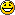Tailhook Chief Captain

Flattery will get you anywhere and everything.

I'm on a real high here... I've actually managed to be of help to someone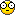Thank you Smailey!

I can't get over it, a complete stranger actually understood what I meant to convey and succeeded with MY help to fix the problemDamn... I shouldn't have had that bottle of SMIRNOFF before I read your post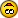...these two very different kind of HIGHS are beginning to clash inside my skull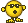All times are GMT Page 1 of 1